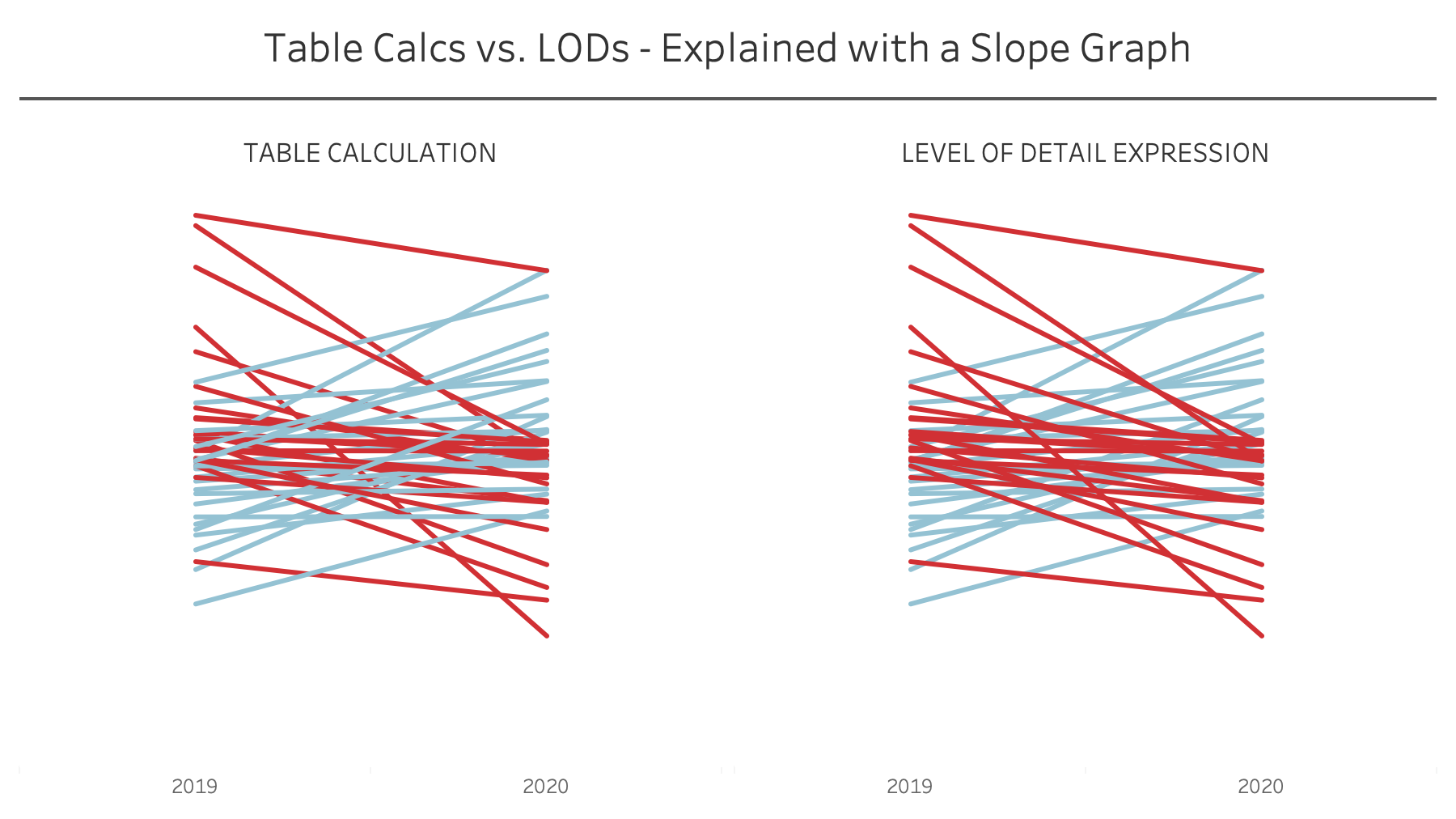## VizWiz

Data Viz Done Right

# Understanding Table Calcs vs LODs: Explained with a Slope GraphTable calculations and LODs, especially understanding the difference between when to use each, is one of the most difficult concepts to learn in Tableau. Through my time teaching, one of the most effective means for explaining the differences between them is with a simple slope graph.

The idea is to color each line of the slope graph by whether it represents an increase or a decrease. You'd think this would be super simple, but it's not. In this video, I show you:

1. How to write the required calculations
2. The benefits of table calcs vs. LODs
3. Why table calcs are often more flexible

My general rule of thumb: If all of the dimensions I need for the calculation I want to write are already in the view, start with a table calculation. If all of the dimensions I need are NOT in the view, then you must use a level of detail expression.